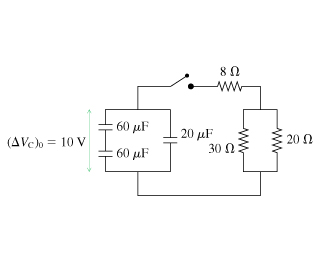# Problem: At what time has the current in the 8 Ω resistor decayed to half the value it had immediately after the switch was closed?

###### FREE Expert Solution

Equivalent resistance for 2 resistors in parallel:

$\overline{){{\mathbf{R}}}_{{\mathbf{eq}}}{\mathbf{=}}\frac{{\mathbf{R}}_{\mathbf{1}}{\mathbf{R}}_{\mathbf{2}}}{{\mathbf{R}}_{\mathbf{1}}\mathbf{+}{\mathbf{R}}_{\mathbf{2}}}}$

Equivalent resistance for resistors in series:

$\overline{){{\mathbf{R}}}_{{\mathbf{eq}}}{\mathbf{=}}{{\mathbf{R}}}_{{\mathbf{1}}}{\mathbf{+}}{{\mathbf{R}}}_{{\mathbf{2}}}{\mathbf{+}}{\mathbf{.}}{\mathbf{.}}{\mathbf{.}}{\mathbf{+}}{{\mathbf{R}}}_{{\mathbf{n}}}}$

For 2 capacitors in series, the equivalent capacitance is::

$\overline{){{\mathbf{C}}}_{\mathbf{e}\mathbf{q}}{\mathbf{=}}\frac{{\mathbf{C}}_{\mathbf{1}}{\mathbf{C}}_{\mathbf{2}}}{{\mathbf{C}}_{\mathbf{1}}\mathbf{+}{\mathbf{C}}_{\mathbf{2}}}}$

For capacitors in parallel, the equivalent capacitance is:

$\overline{){{\mathbf{C}}}_{\mathbf{e}\mathbf{q}}{\mathbf{=}}{{\mathbf{C}}}_{{\mathbf{1}}}{\mathbf{+}}{{\mathbf{C}}}_{{\mathbf{2}}}{\mathbf{+}}{\mathbf{.}}{\mathbf{.}}{\mathbf{.}}{\mathbf{+}}{{\mathbf{C}}}_{{\mathbf{n}}}}$

The decay equation for current:

$\overline{)\begin{array}{rcl}{\mathbf{i}}& {\mathbf{=}}& {\mathbf{i}}_{\mathbf{0}}{\mathbf{e}}^{\mathbf{-}\mathbf{t}}{\mathbf{R}\mathbf{C}}}\end{array}}$

The equivalent resistance:

Req = 8 + (30)(20)/(30 + 20) = 20Ω

88% (71 ratings)###### Problem DetailsAt what time has the current in the 8 Ω resistor decayed to half the value it had immediately after the switch was closed?